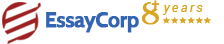WhatsApp
Struggling With Assignments?

We are here to help.

Chat with our expert writers on WhatsApp

for any kind of assignment queries.

Click to chat!
Struggling with Assignments? WhatsApp UsQuestion:

Write a set of hypotheses (null and alternative hypotheses) to answer the question.Show a sampling distribution of the years in college for KU students using R.Submit your R commands, as well.What is the probability of a KU student taking longer than 5.8 year to graduate?

Part I. (OLS) Regression, Sampling Distributions, and Hypothesis Testing (20 points)
There are 30,000 students enrolled in King University (KU). On average, KU students take
5.5 years to graduate (get a bachelor’s degree). The years in college until graduation are
normally distributed with the standard deviation of 0.8.

Mean=5.5
SD=0.8
N=30000
We don’t know the average years in college for KU students majoring in political science, but
we have a random sample of years in college for 16 KU political science students. The
sample mean is 5.8 years.

N=16
Mean= 5.8
Can we state that KU political science students take longer time to graduate than does an
average KU student?

Given Information:
Part I. (OLS) Regression, Sampling Distributions, and Hypothesis Testing (20 points)
There are 30,000 students enrolled in King University (KU). On average, KU students take
5.5 years to graduate (get a bachelor’s degree). The years in college until graduation are
normally distributed with the standard deviation of 0.8.

We don’t know the average years in college for KU students majoring in political science, but
we have a random sample of years in college for 16 KU political science students. The
sample mean is 5.8 years.

Can we state that KU political science students take longer time to graduate than does an
average KU student?
1. Write a set of hypotheses (null and alternative hypotheses) to answer the question.
Null Hypothesis
H0= μ>5.5
Solution:
Xbar= 5.5
.μ= 5.8
N=16
P(X>5.5)
Standard Normal Variate
Z= Xbar- μ/σ
Z= (5.5-5.8)/0.8
Z=-0.3/0.8
Z=-0.375
P(X>5.5)= 1-P(X<5.5)
P(Z>5.5)= 1-P(Z<5.5)
P(Z>5.5)= 1-(-0.375)= 1.375
P(Z>5.5)= 0.9154

Hence associated probability is 0.9154, hence there is no sufficient evidence that KU political
science students take longer time to graduate than does an average KU student.
2.1. Show a sampling distribution of the years in college for KU students using R. Submit your R
commands, as well.

x <- seq(3, 8, length.out = 100)
y1 <- dnorm(x, 5.5, 0.8)
y2 <- dnorm(x, 5.8, 0.8)
plot(y1 ~ x, type = "l", ylab = "Density",
xlab = "Years in college",
main = "Years in college Sampling distribution",
axes = FALSE)
lines(y2 ~ x, col = 2)
legend("topleft",
fill = 1:2,
legend = c("Population distribution", "Sample
distribution"))
axis(1)
axis(2)

HIRE EXPERT
Email ID *
Subject
Pages